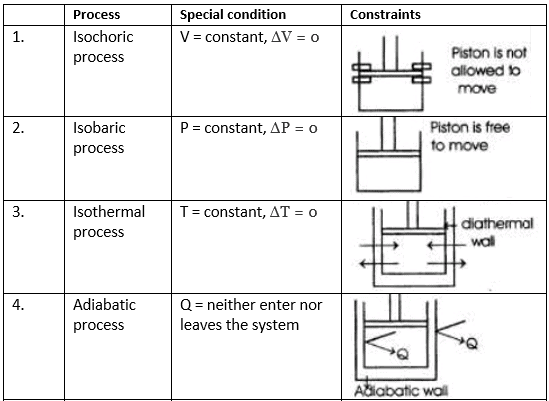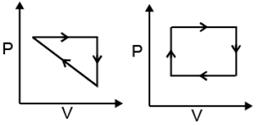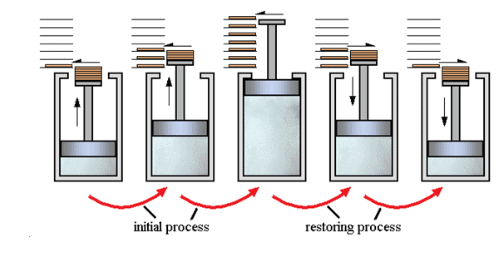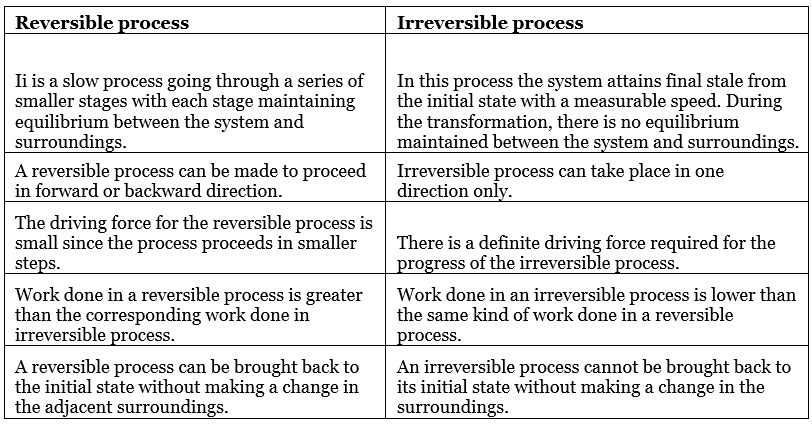Courses

# State of System, Thermodynamic Processes and Internal Energy Class 11 Notes | EduRev

## Class 11 : State of System, Thermodynamic Processes and Internal Energy Class 11 Notes | EduRev

The document State of System, Thermodynamic Processes and Internal Energy Class 11 Notes | EduRev is a part of the Class 11 Course Chemistry for JEE.
All you need of Class 11 at this link: Class 11

TYPES OF EQUILIBRIUM
(i) Thermal equilibrium → Equality of temperature
(ii) Mechanical equilibrium → Equality of pressure
(iii) Material equilibrium → no. of moles constant
When all the three equilibrium are established in a system, system is in true thermodynamics equilibrium.

THERMODYNAMIC PROCESSES
It is an operation by which a system undergoes a change from one state to another.
(i) Isothermal Process: A process in which the temperature remains constant throughout its course is called isothermal process.
i.e. ΔT = 0

(ii) Adiabatic Process: A process during which no heat is exchanged between the system and the surroundings is called adiabatic process.
i.e. dq = 0

(iii) Isobaric Process: A process in which pressure of the system remains constant is called isobaric process.
i.e. ΔP = 0

(iv) Isochoric Process: A process in which volume of the system remains constant is called isochoric process.
i.e. ΔV = 0(v) Cyclic Process: System undergoes series of changes & ultimately comes back to initial state.
i.e., Δ U cyclic = 0; Δ H cyclic = 0REVERSIBLE AND IRREVERSIBLE PROCESSES
Reversible process:

• A process that follows the reversible path, i.e., the process which occurs in infinite number of steps in this Way that the equilibrium conditions are maintained at each step, and the process can be reversed by infinitesimal change in the state of functions.
• If system & surrounding can restore their original state by reversing the direction of the process then process is called reversible process.
• In reversible process, there is no loss of energy.
• These are slow process as it takes infinite time. System & surrounding are always in equilibrium. Reversible process is a theoretical process. Reversible process is most efficient with respect to work.
• In reversible process Pext = PintFig: Reversible & Irreversible Processes

Irreversible process: The process which cannot be reversed and amount of energy increases. All natural processes are Irreversible.

• Irreversible process is a fast process. It takes definite time for completion
• In irreversible process Pext ≠ Pint.
• It is an actual process. It is carried out in multiple stages and it tends towards reversible process.

COMPARISON BETWEEN REVERSIBLE AND IRREVERSIBLE PROCESSESINTERNAL ENERGY (E OR U)

• It is the total energy within the substance.
• It is the sum of many types of energies like vibrational energy, translational energy. etc.
• Energy (U) = TE + RE +  VE + Chemical energy + nuclear energy +  electron spin energy + PE
• U of the system, which may change, when
Heat passes into or out of the system,
Work is done on or by the system,
Matter enters or leaves the system.
• It is an extensive property and state function.
• It is the change in internal energy accompanying a chemical or a physical process that is of interest and this is a measurable quantity.
• Its absolute value cannot be determined but experimentally change in internal energy (Δ) can be determined by
• ΔU = U2 – U1 or ΣUP – ΣUR
• Sign Convention:

➢ For exothermic process, ΔU = -ve , whereas
➢ for endothermic process ΔU = +ve

• U or E depends on temperature, pressure, volume and quantity of matter.

Offer running on EduRev: Apply code STAYHOME200 to get INR 200 off on our premium plan EduRev Infinity!

## Chemistry for JEE

223 videos|452 docs|334 tests

,

,

,

,

,

,

,

,

,

,

,

,

,

,

,

,

,

,

,

,

,

,

,

,

;